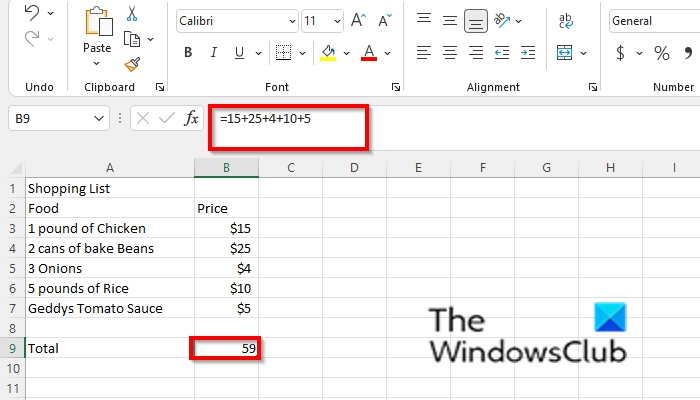# How to create Formula to Add, Subtract, Multiply or Divide in Excel

Microsoft Excel allows users across the globe to perform common and complex calculations, and it helps individuals to complete complicated work or assignments given to them. One of the most important functions of Excel is its capability to perform formulas that assist users to add, multiply, divide, and subtracting numbers.  When a formula is entered into an Excel Spreadsheet, and you have hit the enter key to see the result in the spreadsheet cell, you will see the formula of the calculation in the formula bar, which is a special toolbar at the top of the Excel spreadsheet. Persons will also use the formula bar to edit their calculations. Although Excel has functions to assist you to make a quick addition, division, subtraction, and multiplications, you can also do simple calculations with arithmetic symbols on your keyboard.

## How to create Formula to perform basic calculations in Excel

### How to create Formula to Add in ExcelWhen it comes to the addition of numbers in Excel, you can add two numbers or more using the plus (+) sign between numbers; the equal sign should be before the formula for it to work. Follow the steps below to create a formula that performs addition.

• Launch Excel or open an existing table with numbers to add.
• Type the calculation into the cell you want to place the result, for eg `=15+25+4+10+5`,
• Press the Enter key to see the result.

### How to create Formula to Subtract in ExcelTo create the formula that performs subtraction, follow the same procedure as the addition formula above; the only difference is instead of using the plus (+) sign, you will use the Minus (-) sign, for example, `=150-78`.

### How to create Formula to Multiply in ExcelTo create a formula that performs the multiplication of numbers, you should use the asterisk symbol (*), for example, `=1200 *3`.

### How to create Formula to Divide in ExcelThe formula that performs the division of numbers is the same procedure as the multiplication formula, but it uses a  slash symbol (/), for example, `=12/4`. See the photo below.

### How do you create a formula in Excel without using a Function?

You have to use a cell reference to allow excel to carry out a calculation. Follow the steps below:

1. Click the cell where you want to enter the formula.
2. In the formula bar, type the equal sign and either select the cell that contains the value you want or type the reference of the cells.

### How do I apply a formula to an entire column in Excel?

If you have a particular column that you want to calculate in a spreadsheet, enter the function, then highlight the column you want to include in the row you want to place the result. The column you have selected with the data to calculate the reference will appear in the cell, then press Enter.

### What is basic formula in spreadsheet?

In Microsoft Excel, a formula is an expression that operates on values in a range of cells. These formulas return a result, even when it is an error. Excel formulas allow users to perform calculations such as addition, subtraction, multiplication, and division of numbers.

### What are the 3 common uses for Excel?

The three most common uses for Excel software are to create budgets, produce graphs and charts, and for sorting data. Excel is used in business to forecast future performance, calculate tax, complete basic payroll, produce charts, and calculate revenues.

We hope this tutorial helps you understand how to create basic formula calculations in Excel; if you have questions about the tutorial, let us know in the comments.